# Resources tagged with: Area - triangles, quadrilaterals, compound shapes

Filter by: Content type:
Age range:
Challenge level:

### There are 43 results

Broad Topics > Measuring and calculating with units > Area - triangles, quadrilaterals, compound shapes### Trapezium Four

##### Age 14 to 16Challenge Level

The diagonals of a trapezium divide it into four parts. Can you create a trapezium where three of those parts are equal in area?### Arrowhead

##### Age 14 to 16Challenge Level

The points P, Q, R and S are the midpoints of the edges of a non-convex quadrilateral.What do you notice about the quadrilateral PQRS and its area?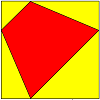### Quadrilaterals in a Square

##### Age 11 to 14Challenge Level

What's special about the area of quadrilaterals drawn in a square?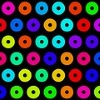### Isometric Areas

##### Age 11 to 14Challenge Level

We usually use squares to measure area, but what if we use triangles instead?### Dividing the Field

##### Age 14 to 16Challenge Level

A farmer has a field which is the shape of a trapezium as illustrated below. To increase his profits he wishes to grow two different crops. To do this he would like to divide the field into two. . . .### From All Corners

##### Age 14 to 16Challenge Level

Straight lines are drawn from each corner of a square to the mid points of the opposite sides. Express the area of the octagon that is formed at the centre as a fraction of the area of the square.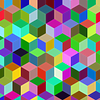### More Isometric Areas

##### Age 11 to 14Challenge Level

Isometric Areas explored areas of parallelograms in triangular units. Here we explore areas of triangles...### Quad in Quad

##### Age 14 to 16Challenge Level

Join the midpoints of a quadrilateral to get a new quadrilateral. What is special about it?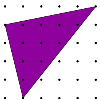### Triangles in a Square

##### Age 11 to 14Challenge Level

What are the possible areas of triangles drawn in a square?### Triangle Transformation

##### Age 7 to 14Challenge Level

Start with a triangle. Can you cut it up to make a rectangle?### Equilateral Areas

##### Age 14 to 16Challenge Level

ABC and DEF are equilateral triangles of side 3 and 4 respectively. Construct an equilateral triangle whose area is the sum of the area of ABC and DEF.### Isosceles

##### Age 11 to 14Challenge Level

Prove that a triangle with sides of length 5, 5 and 6 has the same area as a triangle with sides of length 5, 5 and 8. Find other pairs of non-congruent isosceles triangles which have equal areas.### Inscribed in a Circle

##### Age 14 to 16Challenge Level

The area of a square inscribed in a circle with a unit radius is, satisfyingly, 2. What is the area of a regular hexagon inscribed in a circle with a unit radius?### Rhombus in Rectangle

##### Age 14 to 16Challenge Level

Take any rectangle ABCD such that AB > BC. The point P is on AB and Q is on CD. Show that there is exactly one position of P and Q such that APCQ is a rhombus.### Bicentric Quadrilaterals

##### Age 14 to 16Challenge Level

Investigate the properties of quadrilaterals which can be drawn with a circle just touching each side and another circle just touching each vertex.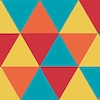### Triangle in a Trapezium

##### Age 11 to 16Challenge Level

Can you find and prove the relationship between the area of a trapezium and the area of a triangle constructed within it?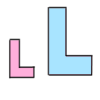### Growing Rectangles

##### Age 11 to 14Challenge Level

What happens to the area and volume of 2D and 3D shapes when you enlarge them?### Kissing Triangles

##### Age 11 to 14Challenge Level

Determine the total shaded area of the 'kissing triangles'.### Six Discs

##### Age 14 to 16Challenge Level

Six circular discs are packed in different-shaped boxes so that the discs touch their neighbours and the sides of the box. Can you put the boxes in order according to the areas of their bases?### Biggest Enclosure

##### Age 14 to 16Challenge Level

Three fences of different lengths form three sides of an enclosure. What arrangement maximises the area?### Overlap

##### Age 11 to 14Challenge Level

A red square and a blue square overlap so that the corner of the red square rests on the centre of the blue square. Show that, whatever the orientation of the red square, it covers a quarter of the. . . .### Squ-areas

##### Age 14 to 16Challenge Level

Three squares are drawn on the sides of a triangle ABC. Their areas are respectively 18 000, 20 000 and 26 000 square centimetres. If the outer vertices of the squares are joined, three more. . . .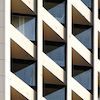### Completing Quadrilaterals

##### Age 11 to 14Challenge Level

We started drawing some quadrilaterals - can you complete them?### At a Glance

##### Age 14 to 16Challenge Level

The area of a regular pentagon looks about twice as a big as the pentangle star drawn within it. Is it?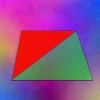### Same Height

##### Age 14 to 16Challenge Level

A trapezium is divided into four triangles by its diagonals. Can you work out the area of the trapezium?### Linkage

##### Age 11 to 14Challenge Level

Four rods, two of length a and two of length b, are linked to form a kite. The linkage is moveable so that the angles change. What is the maximum area of the kite?### Towering Trapeziums

##### Age 14 to 16Challenge Level

Can you find the areas of the trapezia in this sequence?### Gutter

##### Age 14 to 16Challenge Level

Manufacturers need to minimise the amount of material used to make their product. What is the best cross-section for a gutter?### Rati-o

##### Age 11 to 14Challenge Level

Points P, Q, R and S each divide the sides AB, BC, CD and DA respectively in the ratio of 2 : 1. Join the points. What is the area of the parallelogram PQRS in relation to the original rectangle?### Tilted Squares

##### Age 11 to 14Challenge Level

It's easy to work out the areas of most squares that we meet, but what if they were tilted?### Areas of Parallelograms

##### Age 14 to 16Challenge Level

Can you find the area of a parallelogram defined by two vectors?### Doesn't Add Up

##### Age 14 to 16Challenge Level

In this problem we are faced with an apparently easy area problem, but it has gone horribly wrong! What happened?### Shear Magic

##### Age 11 to 14Challenge Level

Explore the area of families of parallelograms and triangles. Can you find rules to work out the areas?### Isosceles Triangles

##### Age 11 to 14Challenge Level

Draw some isosceles triangles with an area of $9$cm$^2$ and a vertex at (20,20). If all the vertices must have whole number coordinates, how many is it possible to draw?### Dotty Triangles

##### Age 11 to 14Challenge Level

Imagine an infinitely large sheet of square dotty paper on which you can draw triangles of any size you wish (providing each vertex is on a dot). What areas is it/is it not possible to draw?### Maths Filler 2

##### Age 14 to 16Challenge Level

Can you draw the height-time chart as this complicated vessel fills with water?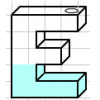### Maths Filler

##### Age 11 to 14Challenge Level

Imagine different shaped vessels being filled. Can you work out what the graphs of the water level should look like?### Kite in a Square

##### Age 14 to 16Challenge Level

Can you make sense of the three methods to work out the area of the kite in the square?### Disappearing Square

##### Age 11 to 14Challenge Level

Do you know how to find the area of a triangle? You can count the squares. What happens if we turn the triangle on end? Press the button and see. Try counting the number of units in the triangle now. . . .### Square Pizza

##### Age 14 to 16Challenge Level

Can you show that you can share a square pizza equally between two people by cutting it four times using vertical, horizontal and diagonal cuts through any point inside the square?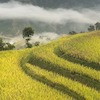### The Farmers' Field Boundary

##### Age 11 to 14Challenge Level

The farmers want to redraw their field boundary but keep the area the same. Can you advise them?### Of All the Areas

##### Age 14 to 16Challenge Level

Can you find a general rule for finding the areas of equilateral triangles drawn on an isometric grid?### Pick's Theorem

##### Age 14 to 16Challenge Level

Polygons drawn on square dotty paper have dots on their perimeter (p) and often internal (i) ones as well. Find a relationship between p, i and the area of the polygons.